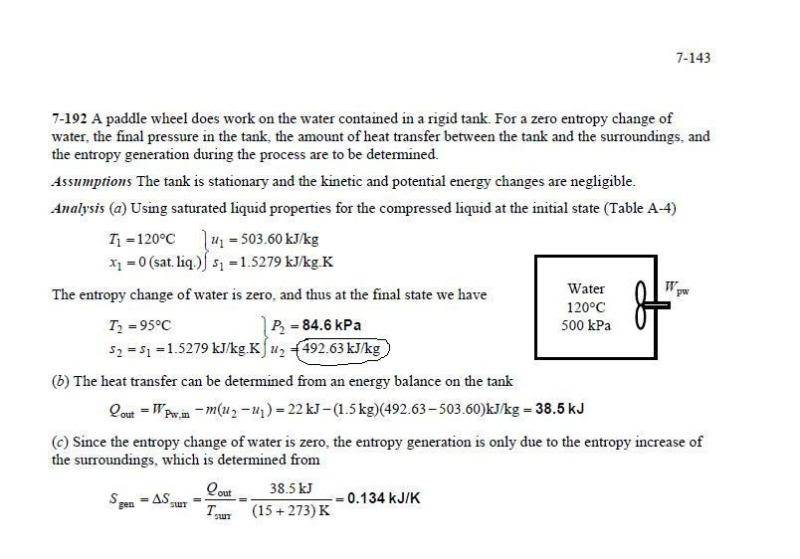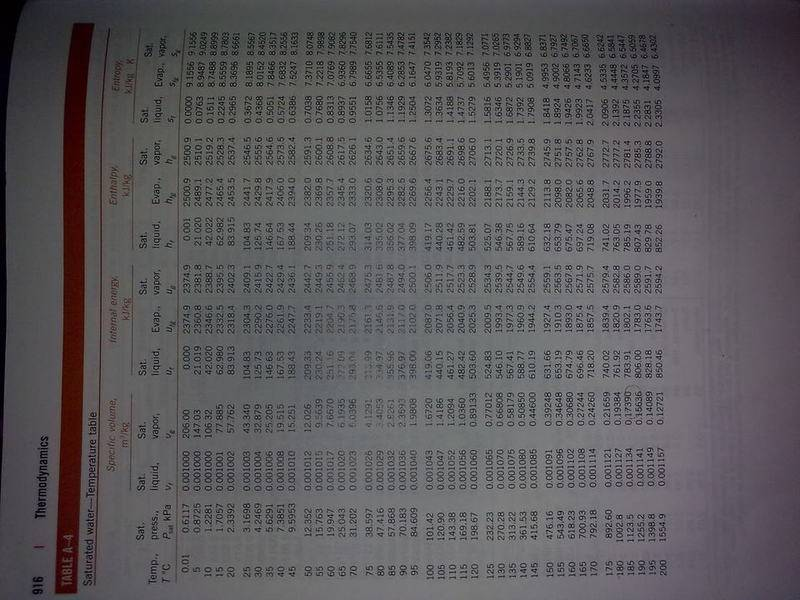# How to determine quality?sorry if i posted this in wrong section..
im having problem on where the value in the circle come from,can someone give me an answer or a hint.
TQLast edited:

Q_Goest
Homework Helper
Gold Member

Hi rojak. This should be in the engineering homework section.

Anyway, do you know how to determine quality? They give you entropy and they tell you the final fluid state is saturated. From the information on entropy and temperature, you should be able to determine the quality. They don't show you how to do that in the example.

Once you have quality, you should be able to determine the internal energy. Again, they don't show you how to do that, but perhaps you can figure it out now.

thank for the reply ^ ^
my english is not so good,here what i understand. find the quality x=(v-vf)/vfg, then substitute it in u=uf+xufg.
im confuse now.. i only have mass of 1.5kg,how do i find the specific volume?
v=V/m
V=?

thanks!! i understand now.. s1=s2..
im using s to find the quality x=(s-sf)/sfg
i got the answer now,thank you very much!